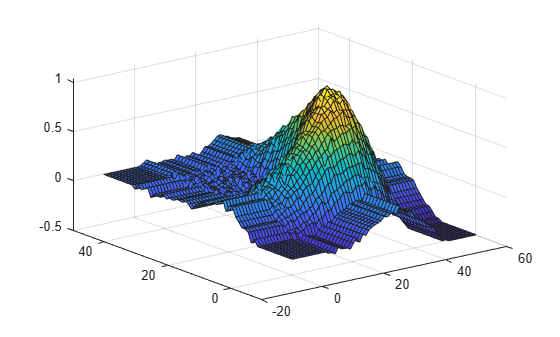## Extrapolation for Interpolant Fit Types

Extrapolation is a process for estimating dependent variable values for independent variable values outside of the fitting data domain. Many different methods perform extrapolation. Given fitting data X for the independent variables and Y for the dependent variable, an extrapolation method creates a function

`$y=f\left(X,Y,u\right),$`

where u is a vector of independent variable values outside of the basic interval, and y is the corresponding estimate for the dependent variable.

Curve Fitting Toolbox™ supports extrapolation for interpolant curve and surface fits. For more information about interpolation, see Interpolation with Curve Fitting Toolbox. The following table describes the supported extrapolation methods in Curve Fitting Toolbox.

Extrapolation MethodDescriptionSupported Interpolation Methods
NoneNo extrapolationLinear, nearest neighbor, and cubic spline for surfaces
Linear

This method fits a linear polynomial at each data point on the boundary of the fitting data's convex hull. Each linear polynomial follows the gradient at the corresponding data point.

Linear, and nearest neighbor and cubic spline for surfaces

Nearest neighbor

This method evaluates to the value of the nearest point on the boundary of the fitting data's convex hull.

Nearest neighbor, and linear and cubic spline for surfaces

Thin-plate spline

This method fits a thin-plate spline through the fitting data and extends it outside of the fitting data's convex hull.

Thin-plate spline

Biharmonic spline

This method fits a biharmonic spline through the fitting data and extends it outside of the fitting data's convex hull.

Biharmonic (v4)

PCHIP

This method fits a shape-preserving piecewise cubic hermite interpolating polynomial (PCHIP) through the fitting data and extends it outside of the fitting data's convex hull.

Shape-preserving (PCHIP)

Cubic spline

This method fits a cubic interpolating spline through the fitting data and extends it outside of the fitting data's convex hull.

Cubic spline for curves

Thin-plate spline extrapolation uses the `tpaps` function, and PCHIP extrapolation uses the `pchip` function. Interpolant surface fits use the MATLAB® function `scatteredInterpolant` function for none, linear, and nearest neighbor extrapolation, and the MATLAB function `griddata` for biharmonic extrapolation.

### Selecting an Extrapolation Method

Curve Fitting Toolbox allows you to choose an extrapolation method for surface fits that use linear, nearest neighbor, or cubic spline interpolation. The extrapolation method you use depends on several factors, including the characteristics of the data being fit, the required smoothness of the curve, and post-fit analysis requirements. You can specify extrapolation methods interactively using the Curve Fitter app, or from the command line using the `fit` and `fitoptions` functions.

#### Select Extrapolation Method Interactively

1. Generate data or load data into the workspace.

2. Open the Curve Fitter app by entering `curveFitter` at the MATLAB command line. Alternatively, on the Apps tab, in the Math, Statistics and Optimization group, click Curve Fitter.

3. In the Curve Fitter app, select curve data. On the Curve Fitter tab, in the Data section, click Select Data. In the Select Fitting Data dialog box, select X data, Y data and Z data.

4. Click the arrow in the Fit Type section to open the gallery, and click Interpolant in the Interpolation group.You can specify the interpolation method by using the Interpolation method menu in the Fit Options pane. If the interpolation method supports multiple extrapolation methods, you can specify the extrapolation method using the Extrapolation method menu.

#### Specify Extrapolation Method from Command Line

Generate some noisy data using the `membrane` and `randn` functions.

```n = 41; M = membrane(1,20)+0.02*randn(n); [X,Y] = meshgrid(1:n);```

The matrix `M` contains data for the L-shaped membrane with added noise. The matrices `X` and `Y` contain the row and column index values, respectively, for the corresponding elements in `M`.

Display a surface plot of the data.

`surf(X,Y,M)`The plot shows a wrinkled L-shaped membrane. The wrinkles in the membrane are caused by the noise in the data.

Specify Extrapolation Method Using `fit` Function

Use the `fit` function to fit a surface through the wrinkled membrane using linear interpolation. Specify the extrapolation method as nearest neighbor.

`linfit = fit([X(:),Y(:)],M(:),"linearinterp",ExtrapolationMethod="nearest")`
``` Linear interpolant: linfit(x,y) = piecewise linear surface computed from p with nearest neighbor extrapolation Coefficients: p = coefficient structure ```

The output confirms that the function uses linear interpolation and nearest neighbor extrapolation to fit a surface through the data.

Evaluate the fit beyond the `X` and `Y` data domain by using the `meshgrid` function. Plot the result using the `surf` function.

```[Xq,Yq] = meshgrid(-10:50); Zlin = linfit(Xq,Yq); surf(Xq,Yq,Zlin);```The plot shows that the nearest neighbor extrapolation method uses the data on the convex hull to extend the surface in each direction. This method of extrapolation generates waves that mimic the convex hull.

Specify Extrapolation Method Using `fitoptions` Function

Use the `fitoptions` function to generate fit options that use nearest neighbor interpolation and linear extrapolation.

`fitOptions = fitoptions("nearestinterp",ExtrapolationMethod="linear")`
```fitOptions = nearestinterpoptions with properties: ExtrapolationMethod: 'linear' Normalize: 'off' Exclude: [] Weights: [] Method: 'NearestInterpolant' ```

`fitOptions` is a fit options object that specifies nearest neighbor interpolation and linear extrapolation.

Fit a surface through the wrinkled membrane using the options in `fitOptions`.

`nearfit = fit([X(:),Y(:)],M(:),"nearestinterp",fitOptions)`
``` Nearest neighbor interpolant: nearfit(x,y) = piecewise constant surface computed from p with linear extrapolation Coefficients: p = coefficient structure ```

Evaluate the fit beyond the `X` and `Y` data domain, and then plot the result.

```Znear = nearfit(Xq,Yq); surf(Xq,Yq,Znear);```The plot shows that the linear extrapolation method generates spikes outside of the `X` and `Y` convex hull. The plane segments that form the spikes follow the gradient at data points on the convex hull border.